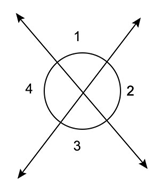# What are Vertically opposite angles and what is their relation?

Vertically Opposite Angles:

When two lines intersect, we get four angles. The angles are two pairs of opposite angels and those angles are known as vertically opposite angles.In the above figure, ∠1, ∠3 and ∠4, ∠2 are vertically opposite angles.

Vertically opposite angles are equal.

∠1 = ∠3 and ∠2 = ∠4.

Updated on: 10-Oct-2022

39 Views